# # 一级标题

## # 二级标题

### # 三级标题

#### # 四级标题

##### # 五级标题
###### # 六级标题
# 一级标题

## 二级标题

### 三级标题

#### 四级标题

##### 五级标题

###### 六级标题


这句话里拥有**加粗**、*倾斜*和~~删除~~


这是一个段落



这是一句话不过我要在这里



> 引用也可以连用
>
> > 可以添加额外的大于号制造更深的引用


• 无序列表项

• 无序列表项

• 列表中的列表项

• 更多的列表项
• 更多的列表项
• 更多的列表项
• 列表中的长列表项，这个列表项很长。

而且由很多个段落构成。

甚至最后一个段落还包含了链接

• 无序列表项

- 无序列表项
- 无序列表项

- 列表中的列表项
- 更多的列表项
- 更多的列表项
- 更多的列表项
- 列表中的长列表项，这个列表项很长。

而且由很多个段落构成。

甚至最后一个段落还包含了[链接](#链接)。

- 无序列表项


1. 有序列表第一项
2. 有序列表第二项
第二项的需要换行
再次换行
3. 有序列表第三项
1. 有序列表第一项
1. 有序列表第二项
第二项的需要换行
再次换行
1. 有序列表第三项


---


[主页](/)


![Logo](/logo.png)


baaaaaaaaaaaaa
caaaaa
|     居中      |         右对齐 | 左对齐         |
| :-----------: | -------------: | :------------- |
| 居中使用:-: | 右对齐使用-: | 左对齐使用:- |
|       b       |      aaaaaaaaa | aaaa           |
|       c       |           aaaa | a              |


行内代码效果: code


var foo = function (bar) {
return bar++;
};

console.log(foo(5));

块级代码:

js
var foo = function (bar) {
return bar++;
};

console.log(foo(5));



## # 上下角标

• 19th
• H2O
- 19^th^
- H~2~O


## # Emoji 表情

😉 😢 😆 😋

:wink: :cry: :laughing: :yum:


😎 😃 :* 😦 😃 😦 😉

8-) :) :\* :( :-) :-( ;)


## # 代码块高亮

<!doctype html>
<html lang="en">
<script>
// Just a lil’ script to show off that inline JS gets highlighted
window.console && console.log('foo');
</script>
<meta charset="utf-8" />
<title>Prism</title>
<script src="assets/vendor/prefixfree.min.js"></script>

<script>
var _gaq = [['_setAccount', 'UA-33746269-1'], ['_trackPageview']];
</script>
<body></body>
</html>

/** @deprecated Use Prism.plugins.fileHighlight.highlight instead. */
Prism.fileHighlight = function () {
if (!logged) {
console.warn(
'Prism.fileHighlight is deprecated. Use Prism.plugins.fileHighlight.highlight instead.',
);
logged = true;
}
Prism.plugins.fileHighlight.highlight.apply(this, arguments);
};

@import url(https://fonts.googleapis.com/css?family=Questrial);

@font-face {
src: url(https://lea.verou.me/logo.otf);
font-family: 'LeaVerou';
}

/*
Shared styles
*/

section h1,
#features li strong,
footer p {
font:
100% Rockwell,
Arvo,
serif;
}


## # 数学公式

Euler’s identity ${e}^{i\pi }+1=0$ is a beautiful formula in ${\mathbb{R}}^{2}$.

Euler’s identity $e^{i\pi}+1=0$ is a beautiful formula in $\mathbb{R}^2$.

$\frac{{\partial }^{r}}{\partial {\omega }^{r}}\left(\frac{{y}^{\omega }}{\omega }\right)=\left(\frac{{y}^{\omega }}{\omega }\right)\left\{\left(\mathrm{log}y{\right)}^{r}+\sum _{i=1}^{r}\frac{\left(-1{\right)}^{i}r\cdots \left(r-i+1\right)\left(\mathrm{log}y{\right)}^{r-i}}{{\omega }^{i}}\right\}$
$$\frac {\partial^r} {\partial \omega^r} \left(\frac {y^{\omega}} {\omega}\right) = \left(\frac {y^{\omega}} {\omega}\right) \left\{(\log y)^r + \sum_{i=1}^r \frac {(-1)^i r \cdots (r-i+1) (\log y)^{r-i}} {\omega^i} \right\}$$


## # 嵌入 HTML

•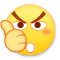0
•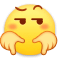0
•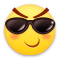0
•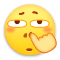0
•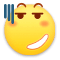0
•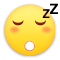0

• 按正序
• 按倒序
• 按热度